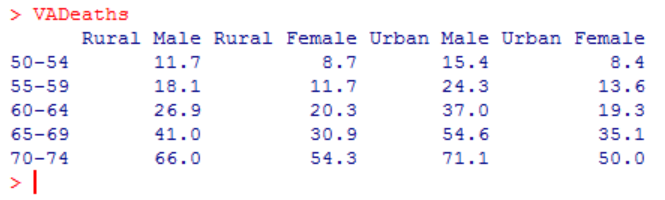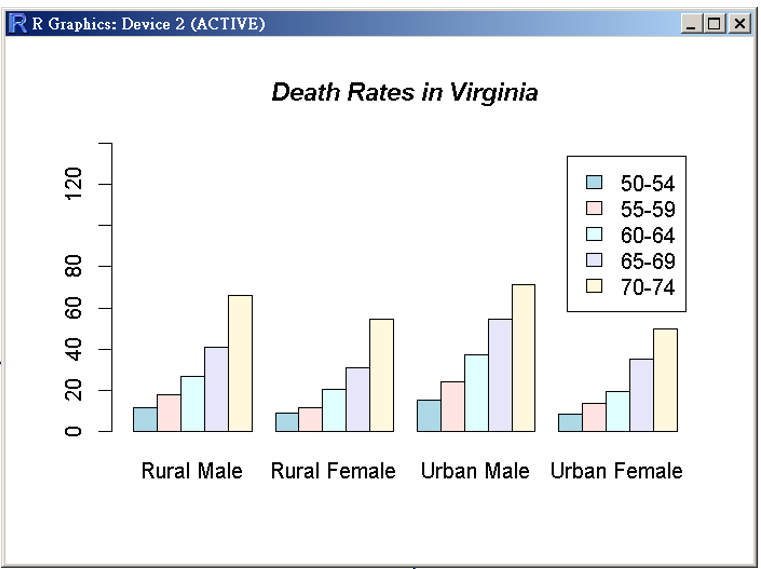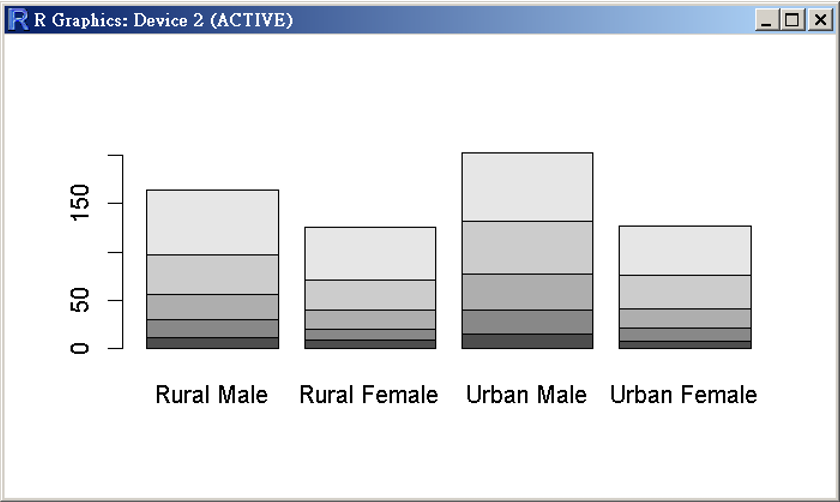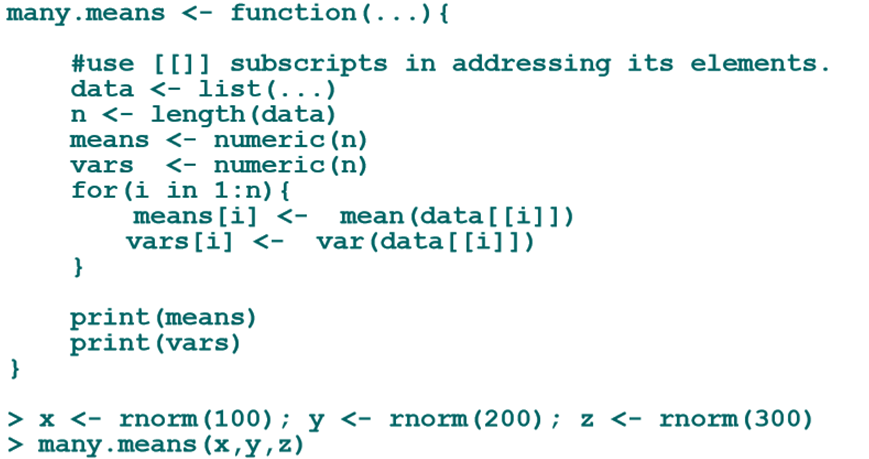0

求救急需幫忙

1. Assume there are 45 students in a class of a high school. They all take 5 courses, namely, calculus, Linear algebra, Management, R programing, and English. The scores of the students are generated by the following R codes:
student.id <- paste(“student”, 1:45, sep = “.”)
set.seed(123)
calculus <- round(rnorm(length(student.id), mean = 65, sd = 10), 0)
linearAlgebra <- sample(1:100, length(student.id), replace = TRUE)
management <- sample(1:100, length(student.id), replace = TRUE)
RProgramming <- sample(1:100, length(student.id), replace = TRUE)
english <- sample(1:100, length(student.id), replace = TRUE)
(a) Please file the students’ ID and scores in an excel file;
(b) Please sort the total scores, consisted of five courses, and then put the result in an excel file.
Note: You need to capture the screen of the excel software to show you reach the desired.
2. In R, there is embedded a data set VADeaths; you can type the following instruction to R and get the following reply.The data set is designed for story for the death rates in Virginia (1940). The death rates are measured per 1000 population per year. They are cross-classified by age group (each row in the above graph) and population group (each column in the above graph).
(a) Please draw the following two graphs
(b) What else you can analyze for the data set? Please suggest and do it.3. Here is a function that takes any number of vectors. Please interpret the meaning of the following codes in your own language.yesongow iT邦大師 1 級 ‧ 2021-06-03 17:40:39 檢舉

pis520 iT邦新手 2 級 ‧ 2021-06-04 07:22:16 檢舉

1 個回答

0

guessvic iT邦新手 4 級 ‧ 2021-06-04 11:24:27 檢舉